## 多维查询

``SELECT * FROM search_table WHERE gender='M' AND city='杭州' OR city='北京';``

``SELECT * FROM search_table WHERE age > 30 AND city != '杭州';``

## 排序翻页

• 搜索索引支持通过`limit`或者`offset`方式进行数据翻页，执行以下语句实现数据翻页查询。
``SELECT * FROM search_table  WHERE gender='M' LIMIT 1,10;``
查询结果如下：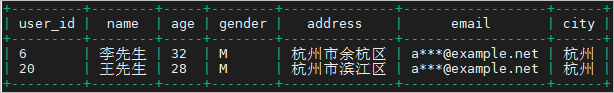• 搜索索引支持任意列的排序，执行以下语句实现数据排序查询。
``SELECT * FROM search_table  WHERE gender='M' ORDER BY age DESC;``
查询结果如下：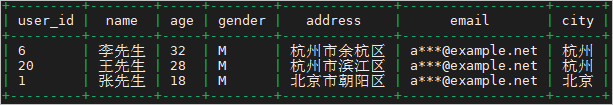## 分词查询

``SELECT * FROM search_table WHERE age > 10 AND age <= 50 AND address='余杭区';``

## 模糊查询

``SELECT * FROM search_table WHERE email LIKE '%example%';``

## 聚合分析

• 执行以下语句，查询`city``杭州`的数据的总数。
``SELECT COUNT(*) FROM search_table WHERE city='杭州';``
查询结果如下：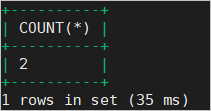• 执行以下语句，实现数据统计去重查询。
``SELECT distinct(age) FROM search_table WHERE gender='M';``
查询结果如下：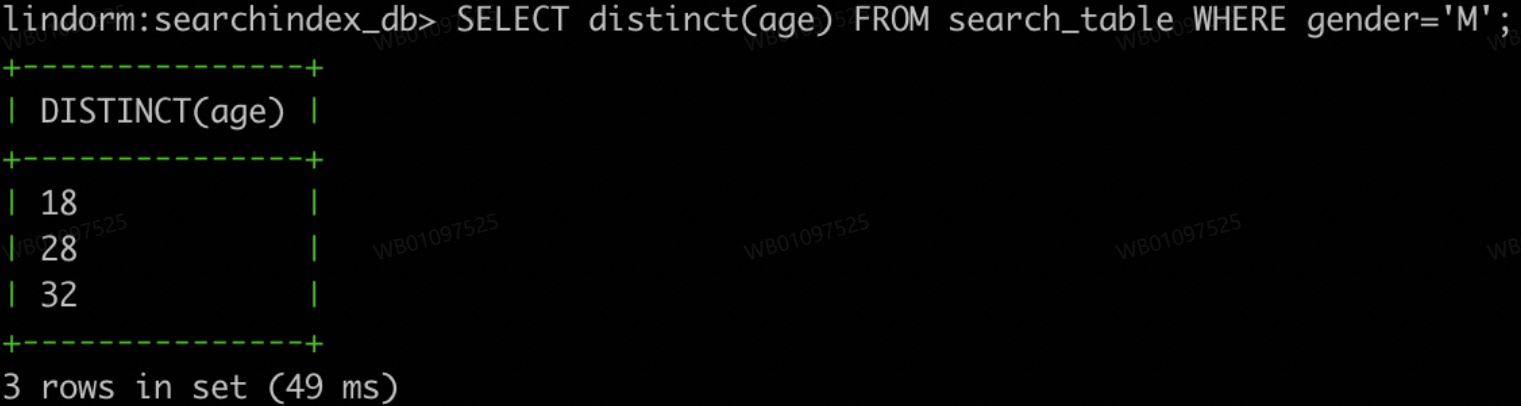• 执行以下语句，实现分组查询。
``SELECT city,count(*) as cnt FROM search_table WHERE gender='M' GROUP BY city ORDER BY cnt DESC;``
查询结果如下：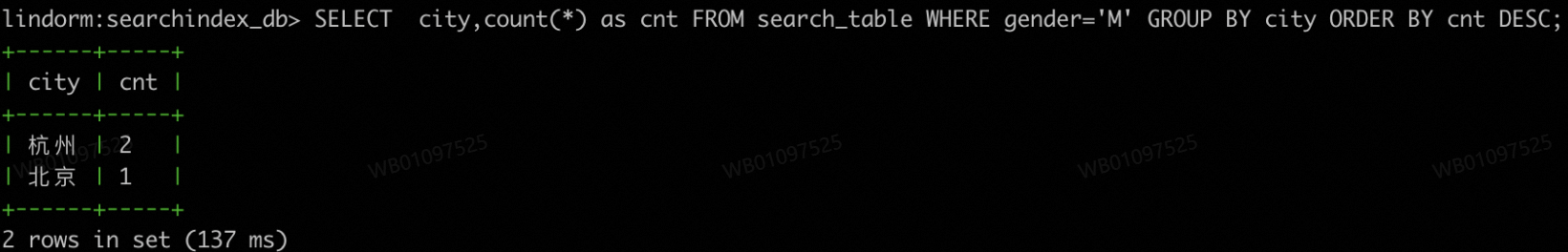## 短语匹配查询

``SELECT name,age,city,address FROM search_table WHERE age > 1 AND age < 100 AND address = '"北京朝阳"' ;``